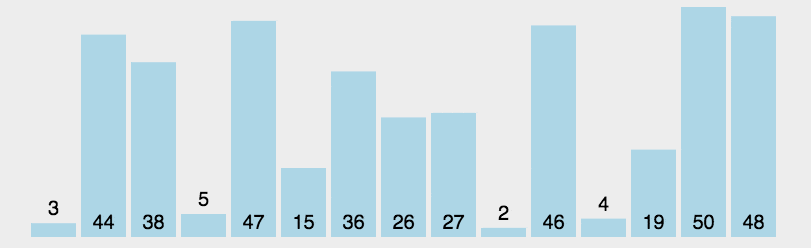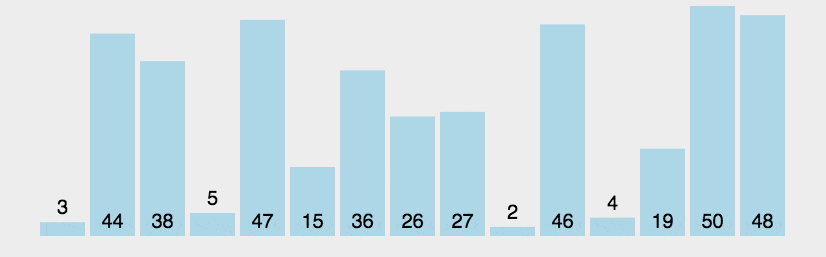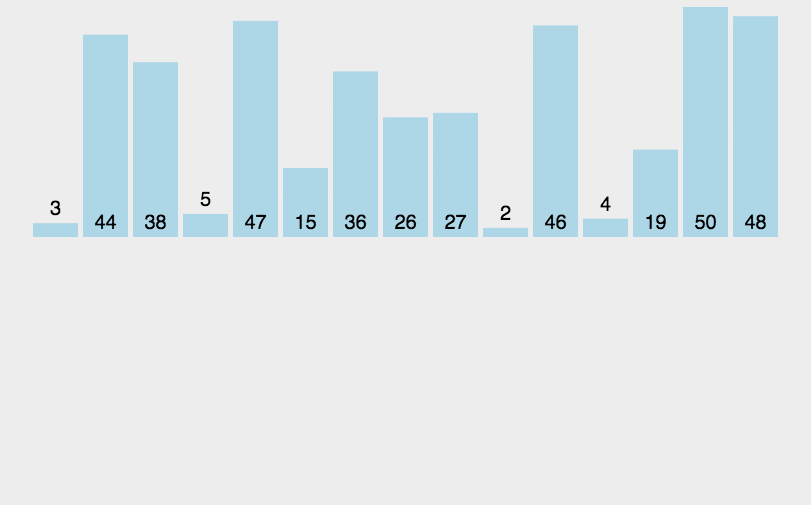Dream,Don't stop a day of hard and don't give up a little hope。 ——不停止一日努力&&不放弃一点希望。

• 累计撰写 32 篇文章
• 累计创建 18 个标签
• 累计收到 10 条评论

### 目 录CONTENT# 排序算法——选择排序、冒泡排序、插入排序 超易理解【JAVA】2022-12-01 / 0 评论 / 0 点赞 / 145 阅读 / 3,008 字

## 一、什么是选择排序算法？

##### 工作原理
• 第一次从待排序的数据元素中选出最小（或最大）的一个元素，存放在序列的起始位置
• 然后再从剩余的未排序元素中寻找到最小（大）元素，然后放到已排序的序列的末尾。
• 以此类推，直到全部待排序的数据元素的个数为零。
##### 图解##### coding
public class 选择排序 {

/**
* 选择排序
* @param arr
*/
public static void selectSort(int[] arr){
//1.先想边界条件 --->arr为空或者小于两个元素，则不用排序
if (arr== null || arr.length<2) {
return;
}
int N = arr.length;
for (int i=0;i<N;i++) {
//0 ~ n-1
//1 ~ n-1
//2 ~ n-1
//i ~ n-1
int minValueIndex = i;  //经过排序后，i的位置就是本轮排序最小值的位置
for (int j=i+1;j<N;j++) {
minValueIndex = arr[j]<arr[minValueIndex] ? j : minValueIndex;
}
swap(arr,i,minValueIndex);
}
}
/**
* 位置交换
*/
public static void swap(int[] arr,int i,int j) {
int tmp = arr[j];
arr[j] = arr[i];
arr[i] = tmp;
}

/**
* 打印数组
* @param arr
*/
public static void printArray(int[] arr) {
for (int i=0;i<arr.length;i++){
System.out.print(arr[i]+" ");
}
System.out.println();
}

public static void main(String[] args) {

int[] arr = {7,1,3,5,1,6,8,1,3,5,7,5,6};
printArray(arr);
selectSort(arr);
printArray(arr);
}
}


## 二、什么是冒泡排序算法？

• 它重复地走访过要排序的数列，每次比较相邻的两个元素，如果他们的顺序错误就把他们交换过来。
• 走访数列的工作是重复地进行直到没有再需要交换，也就是说该数列已经排序完成。

##### 工作原理##### coding
public class 冒泡排序  {

public static void main(String[] args) {

int[] arr = {7,1,3,5,1,6,8,1,3,5,7,5,6};
printArray(arr);
bubbleSort(arr);
printArray(arr);
}

private static void bubbleSort(int[] arr) {
if (arr==null || arr.length<2) {
return;
}
for (int i=0;i<arr.length-1;i++) {
// 设定一个标记，若为true，则表示此次循环没有进行交换，也就是待排序列已经有序，排序已经完成。
boolean flag = true;
for (int j=0;j<arr.length-i-1;j++){
int mid =0;
if (arr[j]>arr[j+1]) {
mid=arr[j];
arr[j] = arr[j+1];
arr[j+1] = mid;

flag = false;
}
}
if (flag) {
break;
}
}
}

private static void printArray(int[] arr) {
for (int i : arr) {
System.out.print(i);
}
System.out.println();
}
}


## 三、什么是插入排序算法？

##### 图解##### coding
public class 插入排序 {

public static void main(String[] args) {

int[] arr = {7,1,3,5,1,6,8,1,3,5,7,5,6};
printArray(arr);
insertSort(arr);
printArray(arr);
}
private static void printArray(int[] arr) {
for (int i : arr) {
System.out.print(i);
}
System.out.println();
}
//插入排序方法一
private static void insertSort(int[] arr) {
if (arr==null || arr.length<2) {
return;
}
// 从下标为1的元素开始选择合适的位置插入，因为下标为0的只有一个元素，默认是有序的
for (int i=1;i<arr.length;i++) {
//记录要插入的数据
int tmp = arr[i];
//从已经排序的序列最右边的开始比较，找到比其小的数
int j = i;
while (j>0 && tmp<arr[j-1]) {
//把要插入的数列拉出来，把它前面的比它小的数列提前
arr[j] = arr[j-1];
j--;
}
if (j != i) {
arr[j] = tmp;
}
}
}

//插入排序方法二
private static void insertZuoSort(int[] arr) {
if (arr==null  || arr.length<2) {
return;
}

for (int i=1;i<arr.length;i++) {
int newNumIndex = i;
while (newNumIndex-1>=0 && arr[newNumIndex]<arr[newNumIndex-1]){
swap(arr,newNumIndex-1,newNumIndex);
newNumIndex--;
}
}
}
//方法三
private static void insertZuoSort2(int[] arr) {
if (arr==null  || arr.length<2) {
return;
}

for (int i=1;i<arr.length;i++) {
//pre 新数的前一个位置
for (int pre = i-1;pre>=0 && arr[pre]>arr[pre+1];pre--) {
swap(arr,pre,pre+1);
}
}
}

private static void swap(int[] arr, int i, int j) {
int tmp=arr[i];
arr[i]=arr[j];
arr[j]=tmp;
}

}


0